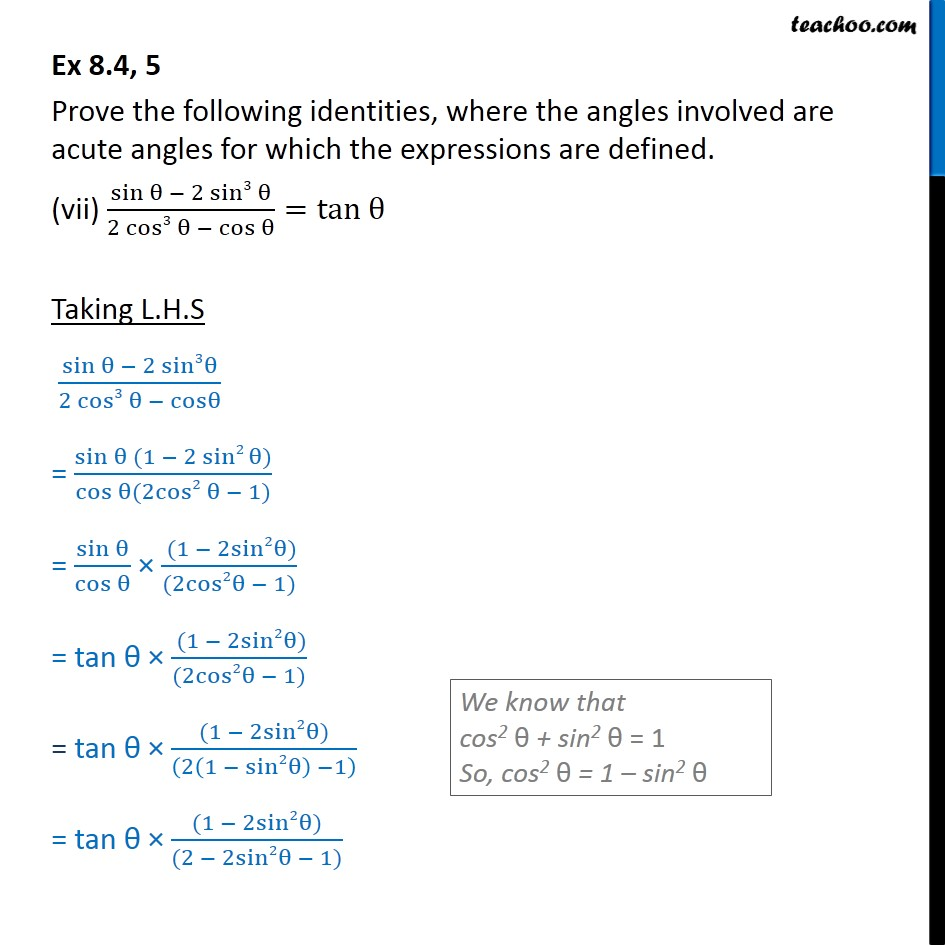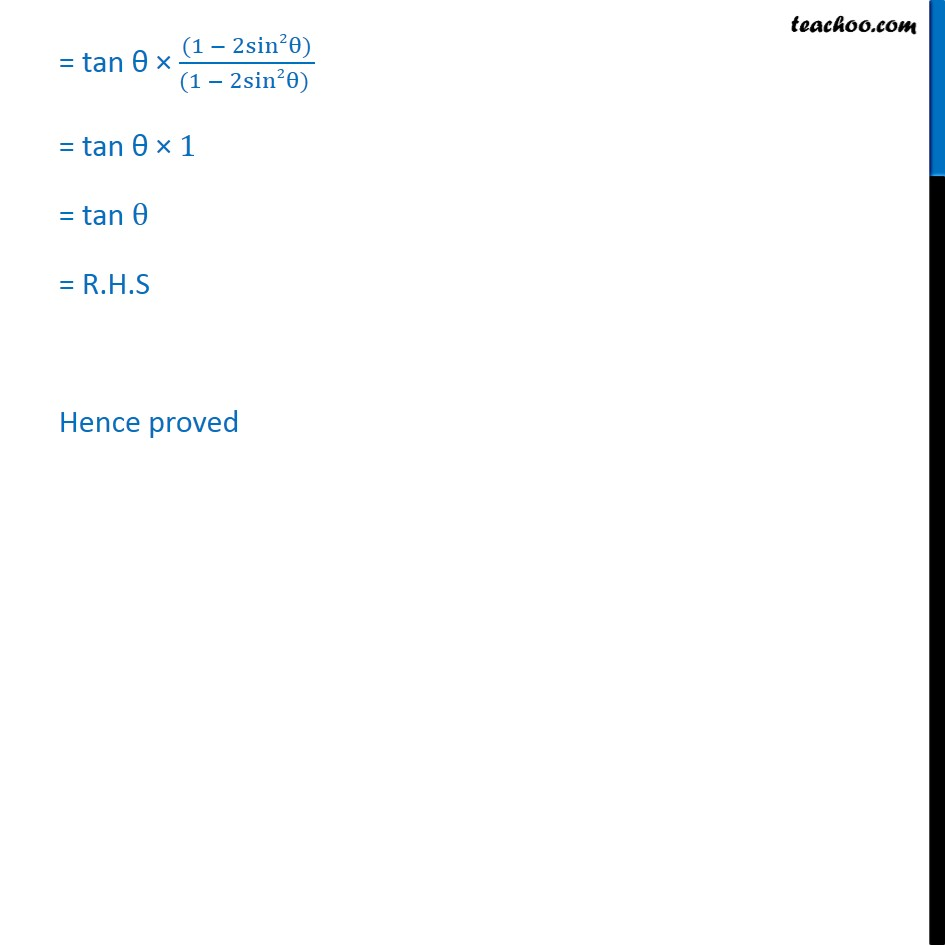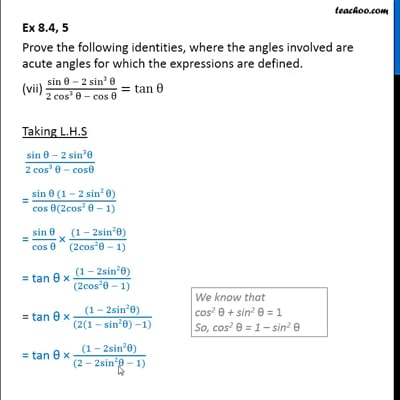Ex 8.4

Chapter 8 Class 10 Introduction to Trignometry
Serial order wiseThis video is only available for Teachoo black users

Introducing your new favourite teacher - Teachoo Black, at only ₹83 per month

### Transcript

Ex 8.4, 5 Prove the following identities, where the angles involved are acute angles for which the expressions are defined. (vii) (sin 2 sin3 )/(2 cos3 cos )=tan Taking L.H.S (sin 2 sin3 )/(2 cos3 cos ) = (sin (1 2 sin2 ))/(cos (2cos2 1)) = sin /(cos ) ( (1 2sin2 ))/((2cos2 1)) = tan ( (1 2sin2 ))/((2cos2 1)) = tan ((1 2sin2 ))/((2(1 sin2 ) 1) ) = tan ((1 2sin2 ))/((2 2sin2 1)) = tan ((1 2sin2 ))/((1 2sin2 ) ) = tan 1 = tan = R.H.S Hence proved